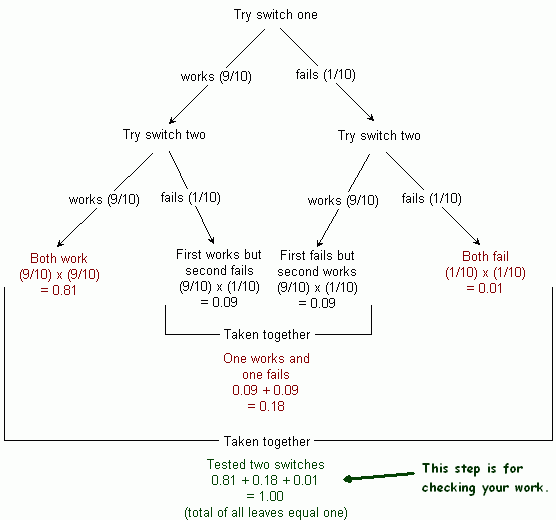SEARCH HOMEMath Central Quandaries & QueriesQuestion from maura, a student: draw a probability to show the outcomes of two new switches (a) of both switches being faulty (b)both switches are not faulty (c) that one switch is faulty. The failure rate is 1/10.Hi Maura.

We can use a probability tree to determine this. Remember that overall probability of each leaf of the tree is obtained by multiplying all its branch probabilities together. Thus, the total probabilities of all the leaves must be equal to one.Hope this helps,
Stephen La Rocque.Math Central is supported by the University of Regina and The Pacific Institute for the Mathematical Sciences.